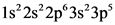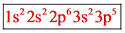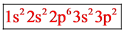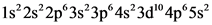# Use the buttons at the top of the tool to add orbitals. click within the orbital to add electrons.

I need help here, I hate thoes creative questions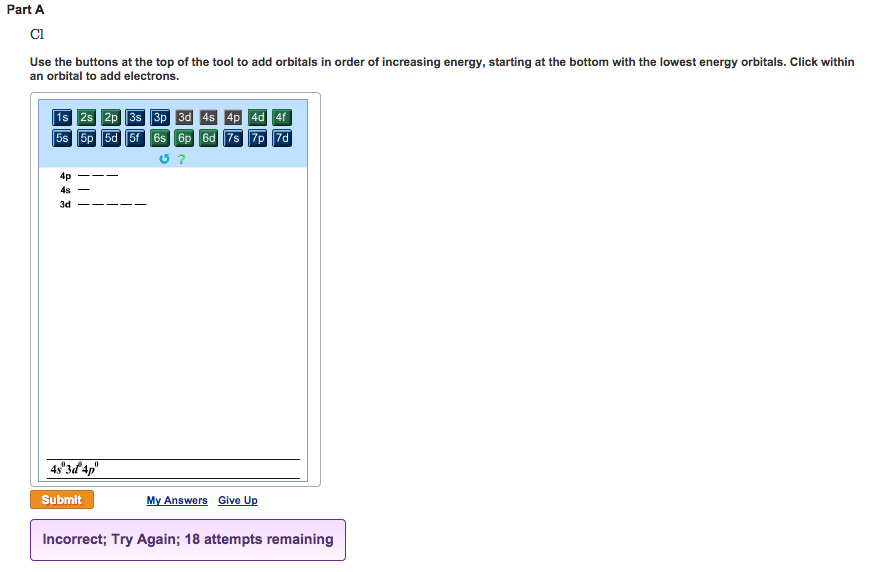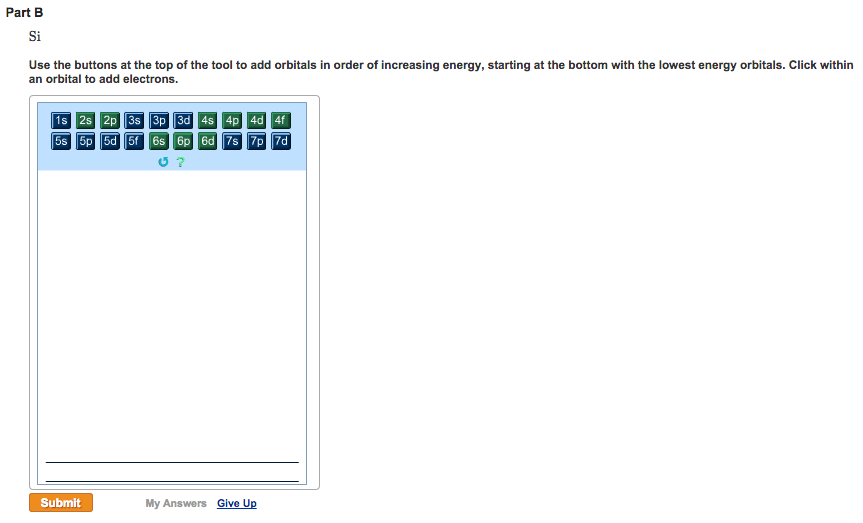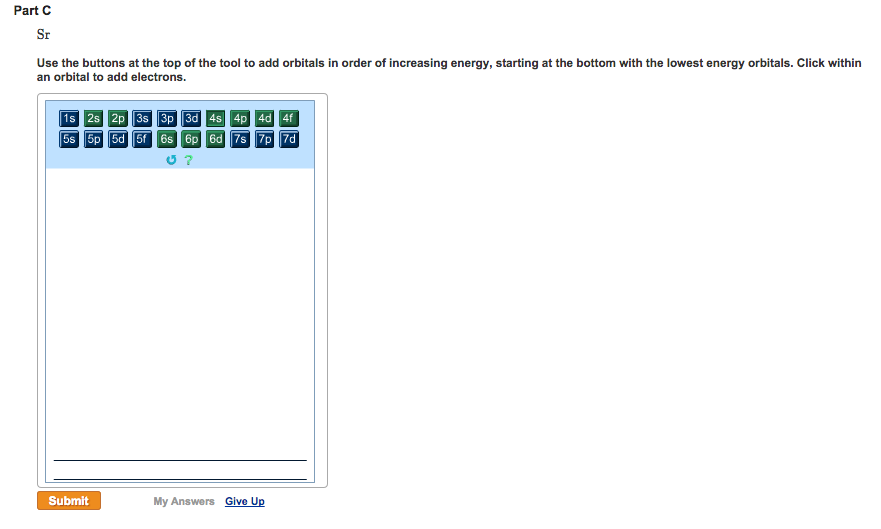Use the buttons at the top of the tool to add orbitals in order of increasing energy, starting at the bottom with the lowest energy orbitals. Click within an orbital to add electrons. Part B Si Use the buttons at the top of the tool to add orbitals in order of increasing energy, starting at the bottom with the lowest energy orbitals. Click within an orbital to add electrons. Part C Sr Use the buttons at the top of the tool to add orbitals in order of increasing energy, starting at the bottom with the lowest energy orbitals. Click within an orbital to add electrons. Part D O Use the buttons at the top of the tool to add orbitals in order of increasing energy, starting at the bottom with the lowest energy orbitals. Click within an orbital to add electrons.

General guidance

Concepts and reason
While filling of electrons in orbitals, we must follow the three rules. They are Aufbau, Hund’s, and Pauli exclusive principle.
According to Aufbau principle, the electrons are in the ground state arranged in orbitals, in the increasing order their energy i.e. energy increasing order from bottom to top.
Hund’s proposed a theory that each orbital filled with one electron, then only pairing possible. If multiple orbitals have the same energy, one electron goes into each of them before they start to double up.
According to Pauli exclusive principle, no two electrons in an atom can have same four quantum numbers.
Therefore, based on these three rules electrons are filling in the orbitals.

Fundamentals

To know the electron filling order for an ion or atom, the atomic number must be known. Thus, based on atomic number of ion or atom, electrons are filling in the orbitals followed by these three rules.
Orbital diagram is as follows:

Step-by-step

Step 1 of 3

(A)
The Given atom is Chlorine (Cl).
The atomic number of Cl atom is 17.
The energy increasing order of orbitals is as follows:
1s, 2s, 2p, 3s, 3p, and so on.
From based on three rules, the orbital filling order of Cl atom is,

Therefore, the orbitals in increasing order of energy for Chlorine atom is .

Part A
Therefore, the orbitals filling order increasing order of energy for Chlorine atom is .

Since, according to Aufbau principle, the electrons are in the ground state arranged in orbitals, in the increasing order their energy i.e. energy increasing order from bottom to top.

Atomic number for an element must write correct. Follow the Aufbau, Hund’s, and pauli exclusive principle.

Consider the given atom and mention the atomic number. Based on atomic number and the available orbitals, electrons are filling from bottom to top. i.e. from low energy orbitals to high energy orbitals.
The given element is Si. It is fourth group element. The number of valence electrons are 4.

Step 2 of 3

(B)
The Given atom is Silicon (Si).
The atomic number of Si atom is 14.
The energy increasing order of orbitals is as follows:
1s, 2s, 2p, 3s, 3p, and so on.
From based on three rules, the orbital filling order of Si atom is,

Therefore, the orbitals in increasing order of energy for Silicon atom is .

Part B
The orbitals filling order in the increasing order of energy for Silicon atom is .

Since, according to Aufbau principle, the electrons are in the ground state arranged in orbitals, in the increasing order their energy i.e. energy increasing order from bottom to top.

Atomic number for an element must write correct. Follow the Aufbau, Hund’s, and pauli exclusive principle.

Consider the given atom and mention the atomic number. Based on atomic number and the available orbitals, electrons are filling from bottom to top. i.e. from low energy orbitals to high energy orbitals.
The given element is Sr. It is second group element. The number of valence electrons are 2.

Step 3 of 3

(C)
The Given atom is Strontium (Sr).
The atomic number of Sr atom is 38.
The energy increasing order of orbitals is as follows:
1s, 2s, 2p, 3s, 3p,4s, 3d,4p, 5s, and so on.
From based on three rules, the orbital filling order of Sr atom is,

Therefore, the orbitals in increasing order of energy for Strontium atom is
.

Part C
The orbitals filling order in the increasing order of energy for Strontium atom is
.

Since, according to Aufbau principle, the electrons are in the ground state arranged in orbitals, in the increasing order their energy i.e. energy increasing order from bottom to top.

Atomic number for an element must write correct. Follow the Aufbau, Hund’s, and pauli exclusive principle.

Part A
Therefore, the orbitals filling order increasing order of energy for Chlorine atom is .

Part B
The orbitals filling order in the increasing order of energy for Silicon atom is .

Part C
The orbitals filling order in the increasing order of energy for Strontium atom is
.

Part A
Therefore, the orbitals filling order increasing order of energy for Chlorine atom is .

Part B
The orbitals filling order in the increasing order of energy for Silicon atom is .

Part C
The orbitals filling order in the increasing order of energy for Strontium atom is
.

AC PL
1s²2s²2pº3s 3p
1s 2s 2p 3s 3p
1s²2s²2pº3s 3p
1s%2s²2pº3s 3p?
1s 2s 2pº3s 3p 4s²3d” 4pº5s2
1s%2s²2p%3s 3pⓇ4s3d” 4p^5s?
1s 2s 2p 3s 3p
1s 2s 2p 3s 3p
1s”2s²2pⓇ3p3s
1s 2s 3s2p 3p
1s 2s 2p 3s 3p
1s%2s²2pº3s 3p?